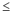# Specify how to estimate the parameters for Zone Chart

Stat > Control Charts > Variables Charts for Subgroups > Zone > Zone Options > Estimate

## Specify subgroups to estimate parameters

If some subgroups have erratic data because of special causes that you have already corrected, you can omit these subgroups from the calculations to avoid incorrect parameter estimates.

1. From the drop-down list, specify whether you want to list the subgroups to omit or the subgroups to include.
• To list the subgroups to omit, select Omit the following subgroups when estimating parameters (eg, 3 12:15).
• To list the subgroups to include, select Use the following subgroups when estimating parameters (eg, 3 12:15).
2. List the subgroups. Use a colon to indicate a range of subgroups. Leave a space between each subgroup or range of subgroups.
For example, to specify subgroups 4, 7, 11, 12, 13, and 14, enter 4 7 11:14.

## Method for estimating standard deviation

Subgroup size = 1
• Average moving range: The average moving range is the average value of the moving range of two or more consecutive points. Use this method when the subgroup size is 1.
• Median moving range: The median moving range is the median value of the moving range of two or more consecutive points. Use this method when the data have extreme ranges that influence average of the moving ranges.
• Square root of MSSD: The square root of MSSD is the square root of the mean of the squared differences between consecutive points. Use this method when you cannot reasonably assume that at least 2 consecutive points were collected under similar conditions.
Subgroup size > 1
• Rbar: Rbar is the average of the subgroup ranges. The Rbar method is a common estimate of the standard deviation and works best with subgroup sizes from 2 to 8.
• Sbar: Sbar is the average of the subgroup standard deviations. The Sbar method provides a more precise estimate of the standard deviation than Rbar, especially with subgroup sizes greater than 8.
• Pooled standard deviation: The pooled standard deviation is the weighted average of subgroup variances, which gives larger subgroups more influence on the overall estimate. The pooled standard deviation method provides a more precise estimate of the standard deviation when the process is in control.

## Specify length of moving range

In Length of moving range, enter the number of observations that are used to calculate the moving range. By default, a length of 2 is used because consecutive values have the greatest chance of being alike. The length must be100.

If the data are cyclical, consider using a moving range of a different length. For example, if you collect quarterly data, consider using a moving range of length 4 to ensure that one observation from each season is included in the calculation.

## Use unbiasing constant

Select Use unbiasing constant to eliminate bias that can occur when you estimate certain process parameters. Often, the choice to use unbiasing constants depends on company policy or industry standards.

By using this site you agree to the use of cookies for analytics and personalized content.  Read our policy